## 6.4    Uniform Circular Motion

From Chapter 4.6 you recall that when a body moves in a circle of radius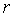with constant speed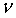, it is said to be in uniform circular motion. The body has a centripetal acceleration of magnitude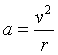directed toward the center of the circle. Applying Newton's second law along the radial direction, one finds that there must be a net centripetal force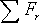of magnitude: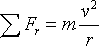to sustain this centripetal acceleration, also directed toward the center of the circle. It is important to recognize that centripetal force is not a new kind of force. The above expression is merely a condition that must be met by whatever force(s) holds the object in this uniform circular motion. In your studies, and in everyday life, you will come across circular motion caused by many different sources such as a normal force, a tension, static friction, gravity, and in later chapters, electric and magnetic forces as well.

In the physlet below is shown a ball (mass = 0.250 kg) attached to a rope whirled in a circular horizontal path (radius = 2.0 m); here the tension in the rope is the centripetal force. Vary the velocity by the slider physlet and observe the effect! Note how the required magnitude of centripetal force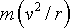is provided by the tension in the rope, and how it is always directed towards the center of the radius.

 Script by Dr. Mohamed S. Kariapper using Physlets from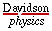Another example is when a car is rounding a curve, the force of friction between the tires and the ground is the centripetal force.

Demos/Animations Examples/Checkpoints Interactive Problems
am = animation ,dm = demo, cp = checkpoint, ex = example, ip = interactive problem